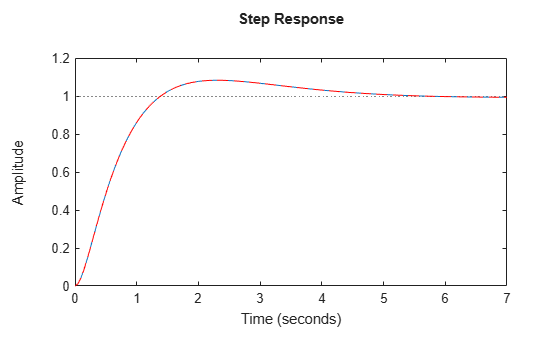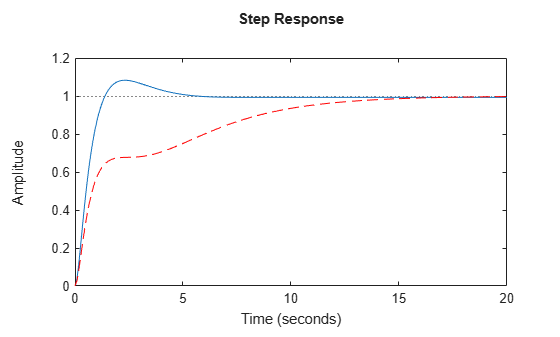# make2DOF

Convert 1-DOF PID controller to 2-DOF controller

## Syntax

``C2 = make2DOF(C1)``
``C2 = make2DOF(C1,b)``
``C2 = make2DOF(C1,b,c)``

## Description

example

````C2 = make2DOF(C1)` converts the one-degree-of-freedom PID controller `C1` to two degrees of freedom. The setpoint weights b and c of the 2-DOF controller are 1, and the remaining PID coefficients do not change. ```
````C2 = make2DOF(C1,b)` specifies the setpoint weight for the proportional term. ```

example

````C2 = make2DOF(C1,b,c)` specifies the setpoint weights for both the proportional and derivative terms. ```

## Examples

collapse all

Design a 1-DOF PID controller for a plant.

```G = tf(1,[1 0.5 0.1]); C1 = pidtune(G,'pidf',1.5)```
```C1 = 1 s Kp + Ki * --- + Kd * -------- s Tf*s+1 with Kp = 1.12, Ki = 0.23, Kd = 1.3, Tf = 0.122 Continuous-time PIDF controller in parallel form. ```

Convert the controller to two degrees of freedom.

`C2 = make2DOF(C1)`
```C2 = 1 s u = Kp (b*r-y) + Ki --- (r-y) + Kd -------- (c*r-y) s Tf*s+1 with Kp = 1.12, Ki = 0.23, Kd = 1.3, Tf = 0.122, b = 1, c = 1 Continuous-time 2-DOF PIDF controller in parallel form. ```

The new controller has the same PID gains and filter constant. It also contains new terms involving the setpoint weights `b` and `c`. By default, `b` = `c` = 1. Therefore, in a closed loop with the plant `G`, the 2-DOF controller `C2` yields the same response as `C1`.

```T1 = feedback(G*C1,1); CM = tf(C2); T2 = CM(1)*feedback(G,-CM(2)); stepplot(T1,T2,'r--')```Convert `C1` to a 2-DOF controller with different `b` and `c` values.

`C2_2 = make2DOF(C1,0.5,0.75)`
```C2_2 = 1 s u = Kp (b*r-y) + Ki --- (r-y) + Kd -------- (c*r-y) s Tf*s+1 with Kp = 1.12, Ki = 0.23, Kd = 1.3, Tf = 0.122, b = 0.5, c = 0.75 Continuous-time 2-DOF PIDF controller in parallel form. ```

The PID gains and filter constant are still unchanged, but the setpoint weights now change the closed-loop response.

```CM_2 = tf(C2_2); T2_2 = CM_2(1)*feedback(G,-CM_2(2)); stepplot(T1,T2_2,'r--')```## Input Arguments

collapse all

1-DOF PID controller, specified as a `pid` object or a `pidstd` object.

Setpoint weight on proportional term, specified as a real, nonnegative, finite value. If you do not specify `b`, then `C2` has `b` = 1.

Setpoint weight on derivative term, specified as a real, nonnegative, finite value. If you do not specify `c`, then `C2` has `c` = 1.

## Output Arguments

collapse all

2-DOF PID controller, returned as a `pid2` object or `pidstd2` object. `C2` is in parallel form if `C1` is in parallel form, and standard form if `C1` is in standard form.

For example, suppose `C1` is a continuous-time, parallel-form `pid` controller of the form:

`${C}_{1}={K}_{p}+\frac{{K}_{i}}{s}+\frac{{K}_{d}s}{{T}_{f}s+1}.$`

Then `C2` is a parallel-form 2-DOF `pid2` controller, which has two inputs and one output. The relationship between the inputs, r and y, and the output u of `C2` is given by:

`$u={K}_{p}\left(br-y\right)+\frac{{K}_{i}}{s}\left(r-y\right)+\frac{{K}_{d}s}{{T}_{f}s+1}\left(cr-y\right).$`

The PID gains Kp, Ki, and Kd, and the filter time constant Tf are unchanged. The setpoint weights b and c are specified by the input arguments `b` and `c`, or 1 by default. For more information about 2-DOF PID controllers, see Two-Degree-of-Freedom PID Controllers.

The conversion also preserves the values of the properties `Ts`, `TimeUnit`, ```Sampling Grid```, `IFormula`, and `DFormula`.

## Version History

Introduced in R2015b